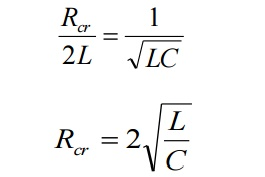Home | | Circuit Theory | The step response of series RLC circuit

# The step response of series RLC circuit

In series RLC circuit, there are two energy storing element which are L and C, such a circuit give rise to second order differential equation and hence called second order circuit.

Details about the step response of series RLC circuitIn series RLC circuit, there are two energy storing element which are L and C, such a circuit give rise to second order differential equation and hence called second order circuit.

Consider a series RLC circuit shown in Figure. The switch is closed at t = 0 and a step voltage of V volts gets applied to circuit.

Apply KVL after switching we getThis is called characteristic equation or auxiliary equation of series RLC circuits.

The response of the circuit depends on the nature of the roots of characteristic equation. The two roots are,Let us define some quantities to find the response according to the nature of roots.

Critical resistance :-

This is the value of resistance which reduces square root term to zero, giving real, equal and negative roots.Damping Ratio (ξ):-

The ratio is the indication of opposition from the circuit to cause oscillations in its response more the value of this ratio, less are the chances of oscillations in the response. It is the ratio of actual resistance in the circuit to critical resistance.

It is   denoted   by   zeta(ξ),3. Natural: (ω)

If the damping is made zero then the response oscillates with natural frequency without any opposition. Such a frequ Frequency of oscillations,.Itisgivenby denoted as ωUsing these values, the roots of equation areThus the response is totally dependent on the Let n α = ξ ωWhere   wd  = actual frequency of oscillations (i.e) damped frequency   when   ξ   =   0,

wdωn (i.e.) natural frequency.

The general solution of characteristic equation isStudy Material, Lecturing Notes, Assignment, Reference, Wiki description explanation, brief detail
Electrical and electronics : Circuit Theory : Resonance and Coupled Circuits : The step response of series RLC circuit |